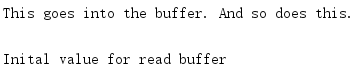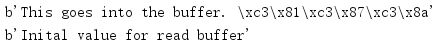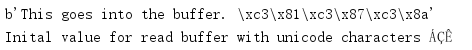# 1. io文本、十进制和原始流I/O工具

io模块在解释器的内置open()之上实现了一些类来完成基于文件的输入和输出操作。这些类得到了适当的分解，从而可以针对不同的用途重新组合——例如，支持向一个网络套接字写Unicode数据。

## 1.1 内存中的流

import io

# Writing to a buffer
output = io.StringIO()
output.write('This goes into the buffer. ')
print('And so does this.', file=output)

# Retrieve the value written
print(output.getvalue())

input = io.StringIO('Inital value for read buffer')

print(input.read())import io

# Writing to a buffer
output = io.BytesIO()
output.write('This goes into the buffer. '.encode('utf-8'))
output.write('ÁÇÊ'.encode('utf-8'))

# Retrieve the value written
print(output.getvalue())

input = io.BytesIO(b'Inital value for read buffer')

print(input.read())## 1.2 为文本数据包装字节流

import io

# Writing to a buffer
output = io.BytesIO()
wrapper = io.TextIOWrapper(
output,
encoding='utf-8',
write_through=True,
)
wrapper.write('This goes into the buffer. ')
wrapper.write('ÁÇÊ')

# Retrieve the value written
print(output.getvalue())

input = io.BytesIO(
b'Inital value for read buffer with unicode characters ' +
'ÁÇÊ'.encode('utf-8')
)
wrapper = io.TextIOWrapper(input, encoding='utf-8')

print(wrapper.read())